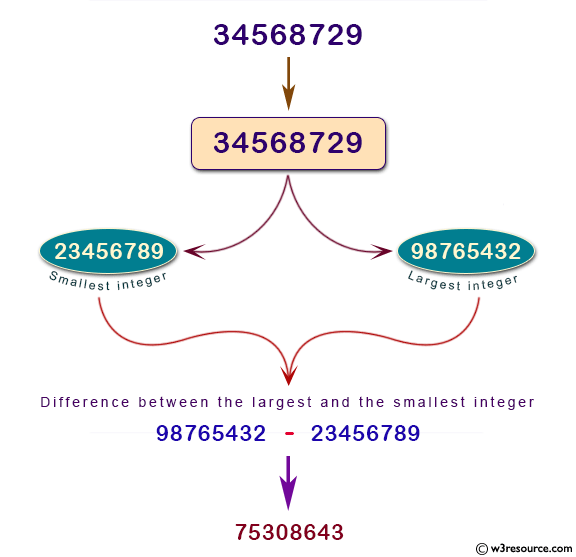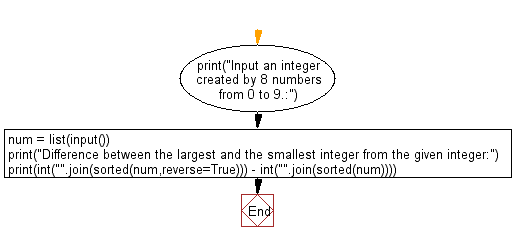﻿ Python: Find the difference between the largest integer and the smallest integer which are created by 8 numbers from 0 to 9 - w3resource

# Python: Find the difference between the largest integer and the smallest integer which are created by 8 numbers from 0 to 9

## Python Basic - 1: Exercise-51 with Solution

Write a Python program that determines the difference between the largest and smallest integers created by 8 numbers from 0 to 9. The number that can be rearranged shall start with 0 as in 00135668.

Input:
Data is a sequence of 8 numbers (numbers from 0 to 9).
Output:
The difference between the largest integer and the smallest integer.

Pictorial Presentation:Sample Solution:

Python Code:

``````print("Input an integer created by 8 numbers from 0 to 9.:")
num = list(input())
print("Difference between the largest and the smallest integer from the given integer:")
print(int("".join(sorted(num,reverse=True))) - int("".join(sorted(num))))
``````

Sample Output:

```Input an integer created by 8 numbers from 0 to 9.:
34568729
Difference between the largest and the smallest integer from the given integer:
75308643
```

Flowchart:Python Code Editor:

Have another way to solve this solution? Contribute your code (and comments) through Disqus.

What is the difficulty level of this exercise?

Test your Programming skills with w3resource's quiz.

﻿